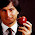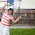Saturday, March 5, 2016

HackerRank: Bear and Steady Gene algorithm (IV)

March 5, 2016

Problem statement:

A gene is represented as a string of length  (where  is divisible by ), composed of the letters , and . It is considered to be steady if each of the four letters occurs exactly  times. For example,  and are both steady genes.

Julia did 3 practice code using C#, but she likes one of solutions using Java, and then, she spent 5 minutes to convert it to C#.

study code:
Java code implementation to study:
https://gist.github.com/jianminchen/d52ced38de0bffa2d9e8

Julia's fourth practice:
use two pointers, sliding window, linear time solution

https://gist.github.com/jianminchen/0892107fc0f6b6bec7a0

Julia's comment:
Julia learned a few things through the code:
1.  Declare cnt array using size of 256, ascii code is 256. So, ACGT shoud be ok.
2.  Declare a string "ACGT", and then the code uses 'A', 'C', 'G', 'T' only once.
3. Only two loops, first loop, variable l - left pointer from 0 to n-1.
second while loop, move r pointer if cnt arrray is bigger than mx - minimum
while (cnt['A'] > mx || cnt['C'] > mx || cnt['T'] > mx || cnt['G'] > mx) {

How to paraphrase this conditional checking?
Let us go through the test case "GAAATAAA",
cnt['A'] = 6, cnt['C'] = 0, cnt['G']= 1, cnt['T'] = 1
mx = 2,
starting from G, and then, while loop <- get in,
G-> GA->...->GAAATA, cnt['A'] = 2, cnt['G'] = 0,

now, it is time to exit while loop. <- making sense. Because cnt array contains the count
for rest of substring "GAAATA", requirement is "none of 'ACGT" is more than 2".

April 14, 2016

I put the for loop into a standalone function, and then, add some comment for the function, explain the design. Basically, it is using sliding window.

Here is the link of code, which passes HackerRank as well.
https://gist.github.com/jianminchen/9b02beab326b2bfcd4b524f219d2946f

statistics:
This post is one of most viewed so far, over 130 views, from March 5 to April 13, 2016.

1.2.